Courses

# Theory Of Random Variable And Noise

## 10 Questions MCQ Test Mock Tests Electronics & Communication Engineering GATE 2020 | Theory Of Random Variable And Noise

Description
This mock test of Theory Of Random Variable And Noise for Electronics and Communication Engineering (ECE) helps you for every Electronics and Communication Engineering (ECE) entrance exam. This contains 10 Multiple Choice Questions for Electronics and Communication Engineering (ECE) Theory Of Random Variable And Noise (mcq) to study with solutions a complete question bank. The solved questions answers in this Theory Of Random Variable And Noise quiz give you a good mix of easy questions and tough questions. Electronics and Communication Engineering (ECE) students definitely take this Theory Of Random Variable And Noise exercise for a better result in the exam. You can find other Theory Of Random Variable And Noise extra questions, long questions & short questions for Electronics and Communication Engineering (ECE) on EduRev as well by searching above.
QUESTION: 1

### A certain random variable has the CDF (cumulative distribution function) given by: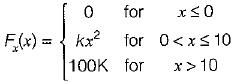Which of the following options is/are not correct?

Solution: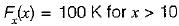Since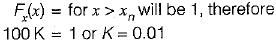Now,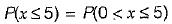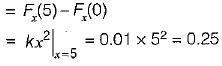Also,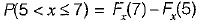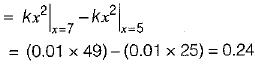Hence, option (c) is not correct.

QUESTION: 2

### A radio receiver with 10 kHz bandwidth has a noise figure of 30 dB. The noise power at input is (take K = 1.38 x 10-23 J/Kelvin and assume room temperature of 300 K)

Solution:

Noise figure,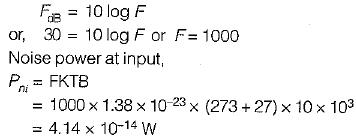QUESTION: 3

### The probability density function of a random variable is given by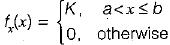The value of constant K is

Solution:

Since the area under the probability density function is equal to 1, therefore.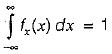or,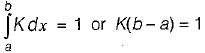or,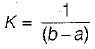QUESTION: 4

Consider the following statements associated with different types of noise occurring in nature:
1. The flicker noise occurs at high operating frequencies (few MHz).
2. Shot noise is produced due to random variations in the arrival of electrons at the output electrode of an amplifying device.
3. The thermal noise is proportional to temperature and the value of the resistance and is independent of bandwidth.
4. Noise can also be represented by fictitious temperature called noise temperature.
Which of the statements given above is/are not correct?

Solution:
• The flicker noise occurs at low operating frequencies (few KHz). Hence, statement-1 is not correct.
• The thermal noise voltage is given by,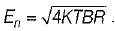Hence, it is proportional to temperature, bandwidth and the value of resistance. Thus, statement-3 is not correct.
• Statements 1 and 4 are correct.
QUESTION: 5

Over a certain binary communication channel (BCC), symbol ‘O' is transmitted with a probability of 0.4 and symbol ‘1’ is transmitted with a probability of 0.6. The probability of error when '1' is transmitted is 10-4 and that when a ‘0' is transmitted is 10-6.
What is the probability of error in the channel?

Solution:

BCC with different probabilities: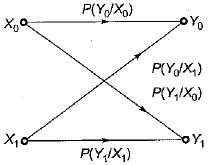According to question,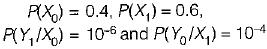Here,  P(Y0/X0) =  Probability of error when ‘1 ’ is transmitted
P(Y1/X0) = Probability of error when ‘0 ’ is transmitted
∴ Probability of error in channel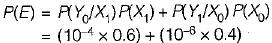or, P(E) = 0.604 x 10-4

QUESTION: 6

Thermal noise is also known as

Solution:

The electronic components generate thermal, shot noise. The thermal noise is also called Johnson noise.

QUESTION: 7

The joint PDF of the.random variable x and y is given by,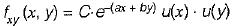where, a and b are constants. The value of C is

Solution:

The joint CDF (cumulative distribution function ) is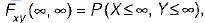which will be equal to 1 because the probability of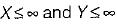covers all the possible values of random variables X and Y.
∴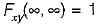According to the definition of CDF, we have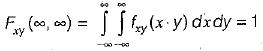Substituting the value of PDF, we have: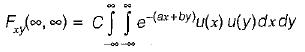or,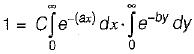or,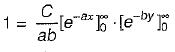or,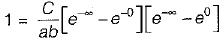or,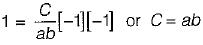QUESTION: 8

Match List-I with List-II and select the correct answer using the codes given below the lists:
List-I
A. Gaussian distribution
B. Probability density function
C. Spectral densities
D. Autocorrelation function
List-II
1. The derivative of cumulative distribution function w.r.t. some dummy variable.
2. A measure of similarity between a signal or process and its replica by a variable amount.
3. Used for continuous random variables.
4. used to represent random process in frequency-domain.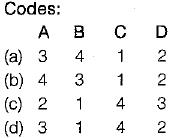Solution:
QUESTION: 9

Assertion (A): if the random process x(t) changes very rapidly with time, the autocorrelation function will decrease rapidly to zero.
Reason (R): The autocorrelation function Rx(τ) is a measure of interdependence of two random variables obtained by observing the random process x(t) at time τ seconds apart.

Solution: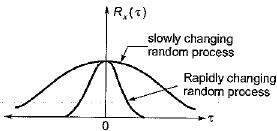From above figure we can conclude that both assertion and reason are true and reason is the correct explanation of assertion.

QUESTION: 10

Consider the following statements associated with conditional probability density function (PDF):
1. The conditional PDF is basically a probability, density function (PDF).
2. The conditional PDF is a non-negative function.
3. The area under a conditional PDF is always equal to 1,
4. The conditional density functions reduce to the marginal density functions.
Which of the statements given above are correct?

Solution:

• The conditional PDF is basically a PDF Since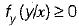i.e. the conditional PDF is a non ­negative function.
Hence, statements 1 and 2 are correct.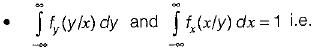th e area under a conditional PDF is always equal to 1. Hence, statement-3 is also correct.
• If the random variables x and y are statistically independent, then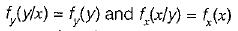This means that the conditional density functions reduce to the marginal density functions. Hence, statement-4 is also correct.